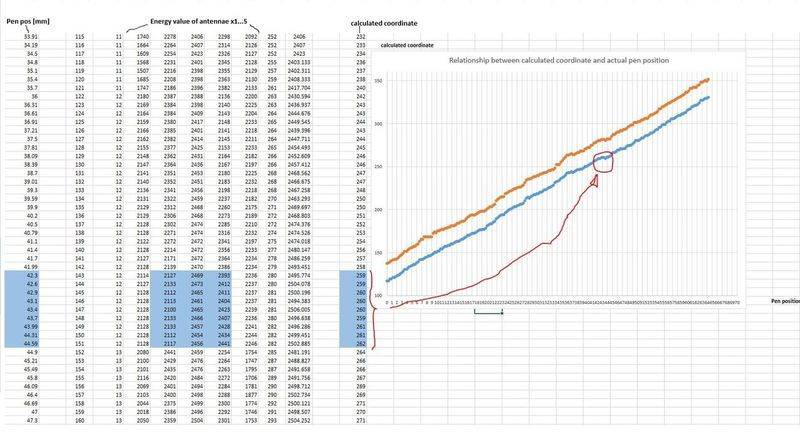# Lagrange interpolation

• I
Nihi
Hi all

I am facing a problem and I hope that you can give me a hand. Here I describe the situation

I am working on a digitizer that can detect the pen position by measuring the antennae energy that are placed in a grid fashion. To get the x coordinate of the pen I measure the energy of three antennae where the pen is supposed to be and then I interpolate this 3 energy-value with Lagrange algorithm.
Finally I determine the pen coordinate by finding the highest f(x) of the curve using a loop.

With Lagrange method it approximates pretty well the actual relationship position-energy but it's not linear.

So here is the problem: when the pen is in the middle of two antennae I get 2 similar energy value whereas the 3rd is lower then these latter, so the highest point of the curve remains about the same when I move the pen around the middle position of 2 antennae. The result is that when the pen crosses these middle points it moves slowly because there is not much changes in the curve which is limited by these 2 similar energy-value.

Is it possible to introduce a factor that changes the curve making it more narrow at the highest point? it's just a thought, I appreciate any solution.In the picture you can see the measurement and the coordinate calculated through Lagrange algorithm. I hope that someone can help me. Thank you!

Hey Nihi.

Have you ever heard of splines before?

Nihi
Hey Nihi.

Have you ever heard of splines before?

Thank you Chiro.
Yes I had. I want to remain with Lagrange and see if I can improve the current result but I will try Spline to see if get better.